Example 62.3 Probit Model with Likelihood Function

The data in this example, taken from Lee (1974), consist of patient characteristics and a variable indicating whether cancer remission occurred. This example demonstrates how to use PROC NLIN with a likelihood function. In this case, twice the negative of the log-likelihood function is to be minimized. This is the objective function for the analysis: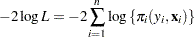In this expression,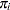denotes the success probability of theBernoulli trials, and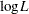is the log likelihood ofindependent binary (Bernoulli) observations. The probabilitydepends on the observations through the linear predictor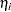,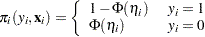The linear predictor takes the form of a regression equation that is linear in the parameters,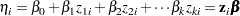Despite this linearity of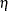in thevariables, the probit model is nonlinear, because the linear predictor appears inside the nonlinear probit function.

In order to use the NLIN procedure to minimize the function, the estimation problem must be cast in terms of a nonlinear least squares problem with objective function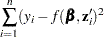This can be accomplished by setting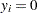and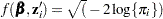. Because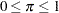, the function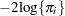is strictly positive and the square root can be taken safely.

The following DATA step creates the data for the probit analysis. The variable like is created in the DATA step, and it contains the value 0 throughout. This variable serves as the "dummy" response variable in the PROC NLIN step. The variable remiss indicates whether cancer remission occurred. It is the binary outcome variable of interest and is used to determine the relevant probability for observationas the success or failure probability of a Bernoulli experiment.

data remiss;
input remiss cell smear infil li blast temp;
label remiss = 'complete remission';
like = 0;
label like = 'dummy variable for nlin';
datalines;
1 0.8  .83 .66 1.9 1.10   .996
1 0.9  .36 .32 1.4 0.74   .992
0 0.8  .88 .70 0.8 0.176  .982
0 1    .87 .87 0.7 1.053  .986
1 0.9  .75 .68 1.3 0.519  .980
0 1    .65 .65 0.6 0.519  .982
1 0.95 .97 .92 1   1.23   .992
0 0.95 .87 .83 1.9 1.354 1.020
0 1    .45 .45 0.8 0.322  .999
0 0.95 .36 .34 0.5 0     1.038
0 0.85 .39 .33 0.7 0.279  .988
0 0.7  .76 .53 1.2 0.146  .982
0 0.8  .46 .37 0.4 0.38  1.006
0 0.2  .39 .08 0.8 0.114  .990
0 1    .90 .90 1.1 1.037  .990
1 1    .84 .84 1.9 2.064 1.020
0 0.65 .42 .27 0.5 0.114 1.014
0 1    .75 .75 1   1.322 1.004
0 0.5  .44 .22 0.6 0.114  .990
1 1    .63 .63 1.1 1.072  .986
0 1    .33 .33 0.4 0.176 1.010
0 0.9  .93 .84 0.6 1.591 1.020
1 1    .58 .58 1   0.531 1.002
0 0.95 .32 .30 1.6 0.886  .988
1 1    .60 .60 1.7 0.964  .990
1 1    .69 .69 0.9 0.398  .986
0 1    .73 .73 0.7 0.398  .986
;

The following NLIN statements fit the probit model:

proc nlin data=remiss method=newton sigsq=1;
parms int=-10 a = -2 b = -1 c=6;

linp = int + a*cell + b*li + c*temp;
p   = probnorm(linp);

if (remiss = 1) then pi = 1-p;
else pi = p;

model.like = sqrt(- 2 * log(pi));
output out=p p=predict;
run;

The assignment to the variable linp creates the linear predictor of the generalized linear model,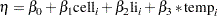In this example, the variables cell, li, and temp are used as regressors.

By default, the NLIN procedure computes the covariance matrix of the parameter estimates based on the nonlinear least squares assumption. That is, the procedure computes the estimate of the residual variance as the mean squared error and uses that to multiply the inverse crossproduct matrix or the inverse Hessian matrix. (See the section Covariance Matrix of Parameter Estimates for details.) In the probit model, there is no residual variance. In addition, standard errors in maximum likelihood estimation are based on the inverse Hessian matrix. The METHOD=NEWTON option in the PROC NLIN statement is used to employ the Hessian matrix in computing the covariance matrix of the parameter estimates. The SIGSQ=1 option replaces the residual variance estimate that PROC NLIN would use by default as a multiplier of the inverse Hessian with the value 1.0.

Output 62.3.1 shows the results of this analysis. The analysis of variance table shows an apparently strange result. The total sum of squares is zero, and the model sum of squares is negative. Recall that the values of the response variable were set to zero and the mean function was constructed asin order for the NLIN procedure to minimize a log-likelihood function in terms of a nonlinear least squares problem. The value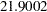shown as the "Error" sum of squares is the value of the function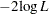.

Output 62.3.1 Nonlinear Least-Squares Analysis from PROC NLIN
The NLIN Procedure

 Note: An intercept was not specified for this model.

Source DF Sum of Squares Mean Square F Value Approx
Pr > F
Model 4 -21.9002 -5.4750 -5.75 .
Error 23 21.9002 0.9522
Uncorrected Total 27 0

Parameter Estimate Approx
Std Error
Approximate 95% Confidence
Limits
int -36.7548 32.3607 -103.7 30.1885
a -5.6298 4.6376 -15.2235 3.9639
b -2.2513 0.9790 -4.2764 -0.2262
c 45.1815 34.9095 -27.0343 117.4

The problem can be more simply solved using dedicated procedures for generalized linear models:

proc glimmix data=remiss;
model remiss = cell li temp / dist=binary link=probit s;
run;

proc genmod data=remiss;
model remiss = cell li temp / dist=bin link=probit;
run;

proc logistic data=remiss;
model remiss = cell li temp / link=probit technique=newton;
run;